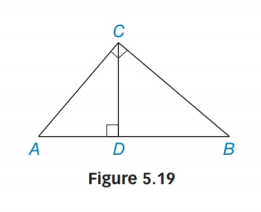Chapter 5.4, Problem 43EElementary Geometry For College St...

7th Edition
Alexander + 2 others
ISBN: 9781337614085

Solutions

Chapter
SectionElementary Geometry For College St...

7th Edition
Alexander + 2 others
ISBN: 9781337614085
Textbook Problem

Use Figure 5.19 to prove Theorem 5.4.2.Theorem 5.4.2The length of the altitude to the hypotenuse of a right triangle is the geometric mean of the lengths of the segments of the hypotenuse.

To determine

To proof :

The theorem 5.4.2, “The length of the altitude to the hypotenuse of a right triangle is the geometric mean of the lengths of the segments of the hypotenuse”.

Explanation

Given:

From the figure ΔABC, with right ACB; CD¯AB¯

Now we have to prove ADCD=CDDB.

Calculation:

 1 ΔABC with right ∠ACBCD¯⊥AB¯ Given 2 CD¯ is an altitude to AB¯ AN altitude is a segment from a vertex and perpendicular the opposite side

Still sussing out bartleby?

Check out a sample textbook solution.

See a sample solution

The Solution to Your Study Problems

Bartleby provides explanations to thousands of textbook problems written by our experts, many with advanced degrees!

Get Started